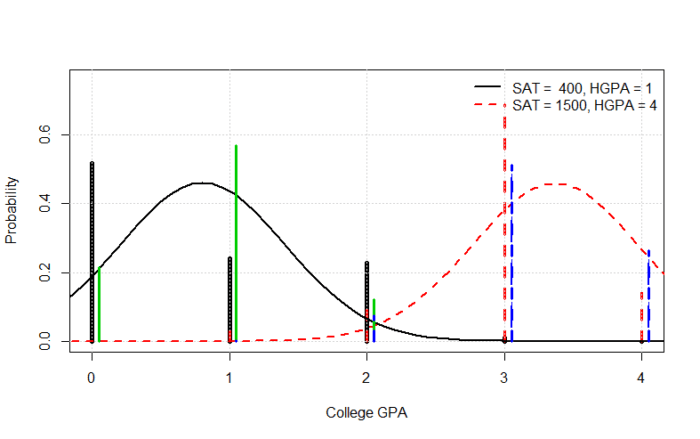Class - Applied Statistics

# Free Probability-Statistics Class: Predictive Case Study 1, Part XI

Review!

We left off with comparing the standard, out-of-the-box linear regression with our multinomial predictive observable model. The great weaknesses of the regression were probability leakage (giving positive probability to impossible values) and that the normal gives densities and not probabilities. We can’t fix the leakage with this model (it’s a built-in shortcoming of this model), but we can generate probabilities.

Now the densities are predictions from the normal regression model, but they are not in a form that can be used. In order to create probabilities from densities, we need to make a decision. The densities are of course easily transformed into cumulative distributions, which are probabilities, but they will give positive probabilities to an infinity of results (all numbers along the continuum). We only care about our decision points, which for our fictional Dean are the five values 0, 1, 2, 3, 4.

The decision we need to make is in how to cut the infinity into 5 blocks. There is of course no unique way to do this. But it may be reasonable to cut the values at the midpoints of the five values. For example, given the regression, the decision probability of having a CGPA = 0 would be the regression probability of being between 0 and 0.05. For 1, it would be 0.5 to 1.5, and so on. That’s easy to do.

Mixing code and output in the obvious way:

```d=as.numeric(levels(x\$cgpa))
caps = d[-length(d)] + diff(d)/2
caps = c(min(d),caps,max(d))

# output
> caps
 0.0 0.5 1.5 2.5 3.5 4.0
```

I used `caps` because R has a native `cut` function inconvenient here (it outputs factors, and we want numbers). Let’s next recreate the picture of the predictions for good and bad students. Then we’ll add on top of it the regression probabilities.

```y = data.frame(cgpa = c("4","4"), sat=c(400,1500), hgpa = c(1,4)) #bad and good student
a=predict(fit, newdata = y, type='prob')\$p #our mulitnomial moels

plot(levels(x\$cgpa), a[1,],type='h',xlab='College GPA',ylab='Probability',ylim=c(0,1.1*max(a)),lwd=5)
lines(levels(x\$cgpa), a[2,],type='h',lty=2,col=2,lwd=3)
grid()
legend('topright', c("SAT =  400, HGPA = 1","SAT = 1500, HGPA = 4"),lty=1:2,col=1:2,bty='n',lwd=2)

s1 = obs.glm(fit.n,y[1,]) # the prediction from the regression
s2 = obs.glm(fit.n,y[2,])

m = s1\$central-s2\$central  # plotting up the probability DENSITIES, NOT PROBABILITIES
s = sqrt(s1\$scale^2+s2\$scale^2)
w = seq(min(s1\$central,s2\$central)-3*s, max(s1\$central,s2\$central)+3*s, length.out = 100)
lines(w,.7*dt((w-s1\$central)/s1\$scale,s1\$df)/s1\$scale,lty=1,lwd=2)
lines(w,.7*dt((w-s2\$central)/s2\$scale,s2\$df)/s2\$scale,lty=2,col=2,lwd=2)

# regression probabilities at the caps
p = matrix(0,2,length(levels(x\$cgpa))) # storage
for (i in 1:2){
b=obs.glm(fit.n,y[i,])
d=pnorm(caps,b\$central,b\$scale) # the predictions
p[i,] = diff(d) # d prob < 0; 1-(sum(diff(d))+d) prob >4
lines(as.numeric(levels(x\$cgpa))+.05,p[i,],col=i+2,type='h',lty=i,lwd=3)
}

```Green solid is for the bad student, regression model; blue dashed is for the good student, regression model. You can see the spikes follow, more or less, the densities. The black solid is our original multinomial model for the bad student, and the red dashed the good student.

Which model is better? There is no way to tell, not with this plot. They are simply two different predictions from two different models. The only way to gauge goodness is to—all together now—wait for new data which has never been seen or used in any way, and then compare both models’ predictions against what happened.

Nevertheless, we can gather clues and build another, different predictive model, which predicts how well our original models will perform. Be sure you understand the distinction! We have an original predictive model. Then we create a model on how well this original predictive model will perform. These are not the same models! This right here is another major departure of the predictive method over classical counterparts.

Let’s first look at the regression predictions a little more closely (mixing code and output):

```> p
[,1]        [,2]       [,3]        [,4]         [,5]
[1,] 2.152882e-01 0.568404758 0.12265228 0.002511169 4.024610e-06
[2,] 1.109019e-06 0.001036698 0.07554629 0.511387677 2.646423e-01

> rowSums(p)
 0.9088604 0.8526141
```

The first row is the bad student (low SAT and HGPA) at each of the five CGPAs (here labeled by their matrix notation), and the second the good student (high SAT and HGPA). The `rowSums(p)` gives the total probability of all possibilities. This should be 1 (right?). It isn’t; it is less, and that is because of probability leakage.

You can see that leakage is conditional on the assumptions, just like probability. Leakage isn’t constant. (It also depends on how we define our caps/cut points.) We earlier guessed, looking only at the densities, that it wasn’t that bad. But it turns out to be pretty large. We’re missing about 10% probability from the first prediction, and about 15% from the second. These are the probabilities for impossible events.

The leakage does not mean the model is useless, but it is evidence the model is sub optimal. It also does not mean our multinomial model is better, but at least our multinomial model does not have leakage. The multinomial model, for instance, can give large probabilities to events that did not happen, while the leakage regression model gives decent probabilities to what happened. We’ll have to see.

And that’s what we’ll do next time. It’s too much to try and begin formal verification this week.

Homework Assume the Dean wants to do CGPAs at 0, 0.5, …, 3.5, and 4. Rerun the code from the beginning, and end with the plot seen above, complete with the regression model using the obvious cut points. Notice anything different?